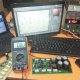Breaking News
Home / how to make inverter / how to make amplifier at home using Battery

# how to make amplifier at home using Battery

## how to make amplifier at home using Battery:

here in this video, we can learn how to make the step-up transformer. the transformer is a way to convert the voltage and the ampere. if you put 100 watts you can get 100 watts. we can’t increase the watts. we can control the volt and ampere only. We know that volt X amp=Watts. also watts/volt = amp. if we use 12 volts 100amp, we can get 1200Watts. we can’t make it more. but we can make 1000 volt or according to our needs. but we can’t cross the watts. if we make 1000 volt we can get a little amp. or if we make 600 voltage we can get 2 amperes. in this article, we are using 12Voltage. and we are making 35 volts. if we use 12 volt 100amp, we can get 35 volts 34 amp max.Normally we can’t use Battery to any high watts amplifier. here we can use a battery to a high watts amplifier. we use a simple inverter to making 12 volts to 35-0-35 voltage. here in this article, we can learn how to make an amplifier at home using Battery. Here we can see that how to make inverter using 12 voltage. we use only, 2 transistors, 2 resistors, and a 12 voltage transformer.

Let’s watch the video, how to make an amplifier at home using Battery

we also can get the diagram. In this Instructable, I will instruct you to make your own 12v DC to 35v AC inverter with less number of components. Inverters are often needed at places where it is not possible to get AC supply from the Mains. An inverter circuit is used to convert the DC power to AC power.

transistor circuit diagram of 2sa1943 and 2sc5200

Thanks a lot to be with us. If you want another post then please visit our website.

We have another post for you. like as repairing amplifier,